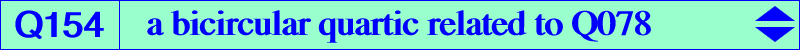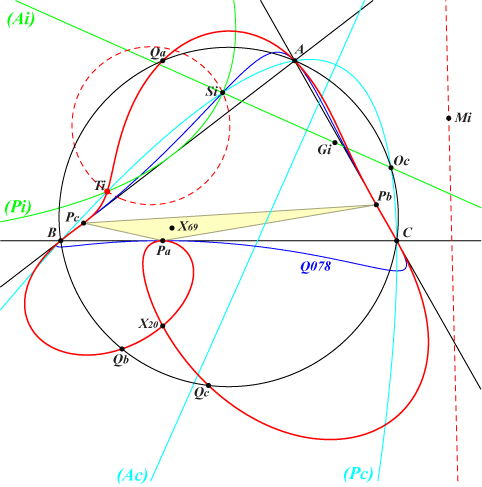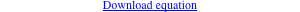too complicated to be written here. Click on the link to download a text file.X(20) common points of (O) and the sidelines of the antimedial triangle namely A, B, C, Qa, Qb, Qc Pa, Pb, Pc : vertices of the cevian triangle of X(69)Two parabolas with parallel axes, one (Pi) inscribed in ABC and one (Pc) circumscribed to ABC, meet at two other isotomic conjugate points M1, M2 lying on K015 such that the line M1M2 is tangent to the Steiner ellipse. When "parallel" is replaced with " perpendicular", the parabolas meet at four points and one of them is the vertex Si of (Pi), a point lying on the bicircular quartic Q078. The three other points lie on another bicircular quartic which is Q154. One of these points Ti is always real. (Pc) meets (O) again at Oc and the centroid Gi of the three points is the image of Oc under the homothety h(Si, 2/3). Note that the circumcircle of the three points passes through Si. Q078 and Q154 must meet at 16 points but in fact, at 8 double points which are A, B, C, Pa, Pb, Pc and the circular points at infinity. It follows that the pencil generated by Q078 and Q154 contains the decomposed quartic which is the union of the sidelines of ABC and the line at infiny. X(20) is a node with tangents parallel to the asymptotes of the circum-conic with perspector X(393), the barycentric square of X(4). This conic contains X(107).